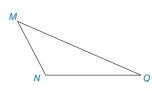Chapter 2.1, Problem 35E### Elementary Geometry for College St...

6th Edition
Daniel C. Alexander + 1 other
ISBN: 9781285195698

#### Solutions

Chapter
Section### Elementary Geometry for College St...

6th Edition
Daniel C. Alexander + 1 other
ISBN: 9781285195698
Textbook Problem
1 views

# Given: Triangle M N Q with obtuse ∠ M N Q Construct: M R ¯ ⊥ N Q ¯ (HINT: Extend N Q ¯ .)Exercise 36, 37

To determine

To construct:

The perpendicular line on the given line.

Explanation

Given:

Triangle MNQ with obtuse MNQ.

The give line is NQ¯.

The given figure is,

Approach:

The given figure is,

Figure (1)

Use compass to make an arc from M that intersect NQ at point A and B as shown in figure (2).

Figure (2)

With A and B as center mark two arcs equal radii MB to intersect at point L as shown in figure (3)

### Still sussing out bartleby?

Check out a sample textbook solution.

See a sample solution

#### The Solution to Your Study Problems

Bartleby provides explanations to thousands of textbook problems written by our experts, many with advanced degrees!

Get Started

#### Find the derivative of the function. g(x)=12x1+2xtsintdt

Single Variable Calculus: Early Transcendentals, Volume I

#### If f(x) 5 for all x [2, 6] then 26f(x)dx. (Choose the best answer.) a) 4 b) 5 c) 20 d) 30

Study Guide for Stewart's Single Variable Calculus: Early Transcendentals, 8th

#### Describe how replication protects against fraud being committed in research.

Research Methods for the Behavioral Sciences (MindTap Course List)